## 9.3.1 Game Formulation

Suppose there are two players,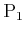and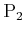, that each have to make a decision. Each has a finite set of actions,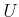and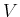, respectively. The setcan be viewed as the replacement'' of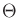from Formulation 9.3 by a set of actions chosen by a true opponent. Each player has a cost function, which is denoted as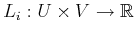for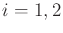. An important constraint for zero-sum games is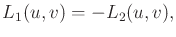(9.41)

which means that a cost for one player is a reward for the other. This is the basis of the term zero sum, which means that the two costs can be added to obtain zero. In zero-sum games the interests of the players are completely opposed. In Section 9.4 this constraint will be lifted to obtain more general games.

In light of (9.41) it is pointless to represent two cost functions. Instead, the superscript will be dropped, and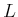will refer to the cost,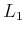, of. The goal ofis to minimize. Due to (9.41), the goal ofis to maximize. Thus,can be considered as a reward for, but a cost for.

A formulation can now be given:

Formulation 9..7 (A Zero-Sum Game)
1. Two players,and.
2. A nonempty, finite setcalled the action space for. For convenience in describing examples, assume thatis a set of consecutive integers fromto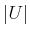. Each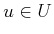is referred to as an action of.
3. A nonempty, finite setcalled the action space for. Assume thatis a set of consecutive integers fromto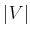. Each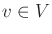is referred to as an action of.
4. A function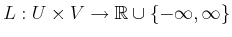called the cost function for. This also serves as a reward function forbecause of (9.41).

Before discussing what it means to solve a zero-sum game, some additional assumptions are needed. Assume that the players know each other's cost functions. This implies that the motivation of the opponent is completely understood. The other assumption is that the players are rational, which means that they will try to obtain the best cost whenever possible.will not choose an action that leads to higher cost when a lower cost action is available. Likewise,will not choose an action that leads to lower cost. Finally, it is assumed that both players make their decisions simultaneously. There is no information regarding the decision ofthat can be exploited by, and vice versa.

Formulation 9.7 is often referred to as a matrix game becausecan be expressed with a cost matrix, as was done in Section 9.2. Here the matrix indicates costs forand, instead of the robot and nature. All of the required information from Formulation 9.7 is specified by a single matrix; therefore, it is a convenient form for expressing zero-sum games.

Example 9..13 (Matrix Representation of a Zero-Sum Game)   Suppose that, the action set for, contains three actions andcontains four actions. There should be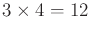values in the specification of the cost function,. This can be expressed as a cost matrix,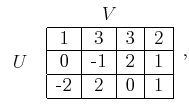(9.42)

in which each row corresponds to some, and each column corresponds to some. Each entry yields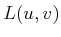, which is the cost for. This representation is similar to that shown in Example 9.8, except that the nature action space,, is replaced by. The cost foris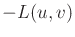.Steven M LaValle 2020-08-14